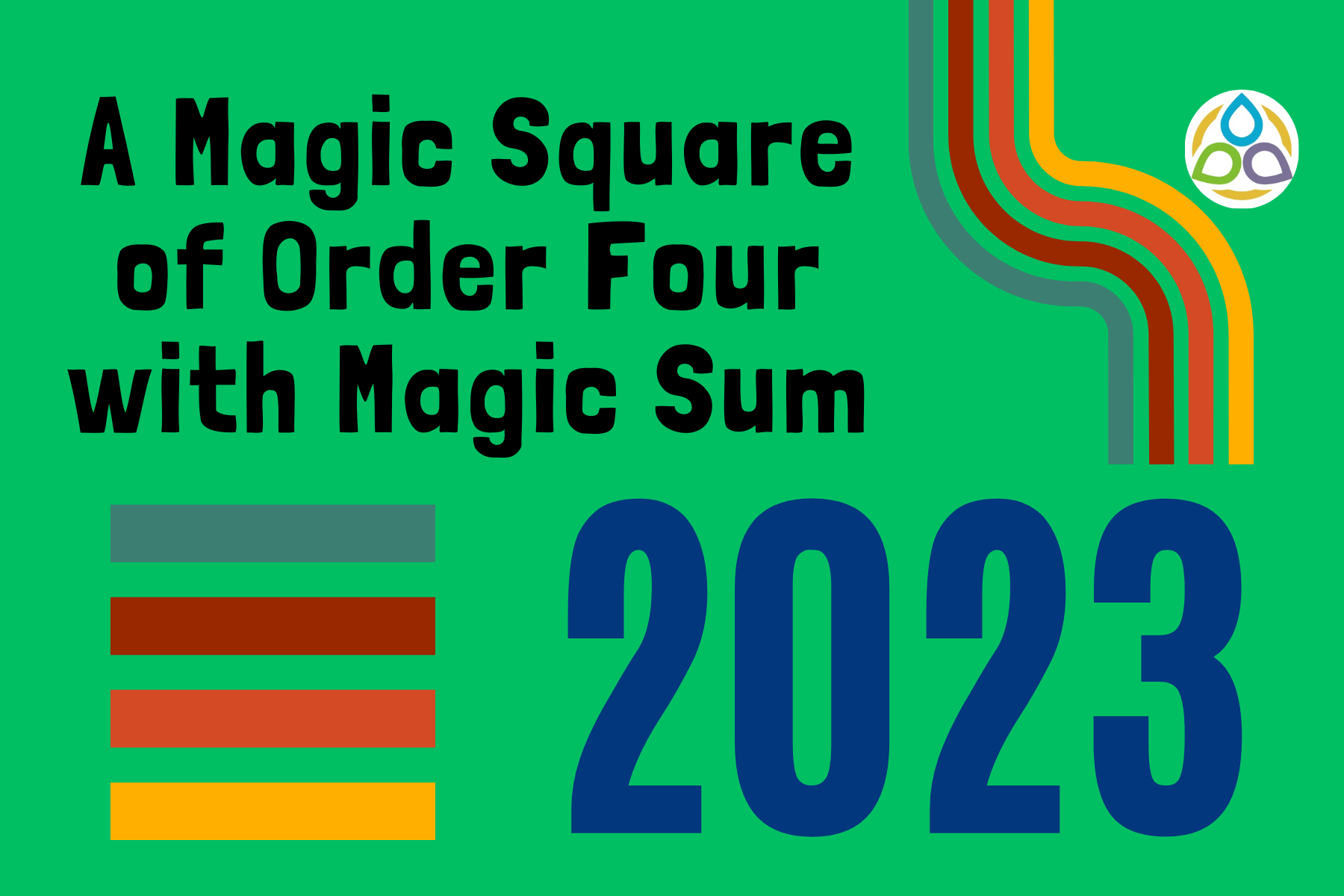## A Magic Square of Order Four with Magic Sum 2023

After exponential quantities, the circular functions, sine and cosine, should be considered
because they arise when imaginary quantities are involved in the exponential.

Leonhard Euler

Welcome to the blog Math1089 – Mathematics for All.

I’m glad you came by. I wanted to let you know I appreciate your spending time here on the blog very much. I do appreciate your taking time out of your busy schedule to check out Math1089!

Consider the following square matrix (same number of rows and columns) number arrangement, which was designed with the current year 2023 in mind. A square arrangement in the form of a matrix becomes a magic square if the following conditions are satisfied:

• (a) The sums along the rows are the same;
• (b) The sums along the columns are the same;
• (c) The sums along the diagonals are the same.

This sum is known as the magic number for that particular magic square.

A square matrix can tell us so many things and even show us some magic. In this blog post, consider the above square matrix with entries 507, 504, 514, 498 etc.

## Few Notable Properties

(a) The sum of the row elements in each row is equal to 2023. For example,

507 + 504 + 514 + 498 = 2023

515 + 497 + 508 + 503 = 2023

500 + 516 + 502 + 505 = 2023

501 + 506 + 499 + 517 = 2023

(b) The sum of the column elements in each column is equal to 2023. For example,

507 + 515 + 500 + 501 = 2023

504 + 497 + 516 + 506 = 2023

514 + 508 + 502 + 499 = 2023

498 + 503 + 505 + 517 = 2023

(c) The sum of the diagonal elements is also equal to 2023. For example,

507 + 497 + 502 + 517 = 2023 (green)

498 + 508 + 516 + 501 = 2023 (light blue)

(d) The sum of the corner elements is also equal to 2023. For example,

507 + 498 + 517 + 501 = 2023

(e) The sums of the numbers in the two sets of like coloured cells are again the same number 2023. For example,

504 + 514 + 499 + 506 = 2023 (yellow)

515 + 500 + 505 + 503 = 2023 (purple)

(f) The sums of the numbers in the two sets of like coloured cells are again the same number 2023. For example,

504 + 515 + 499 + 505 = 2023 (yellow)

514 + 503 + 506 + 500 = 2023 (blue)

(g) The sum of the numbers in the four central cells is again 2023. For example,

497 + 508 + 502 + 516 = 2023

(h) The sums of the numbers in the like-coloured 2 × 2 blocks are all 2023. For example,

507 + 504 + 497 + 515 = 2023 (light blue)

514 + 498 + 503 + 508 = 2023 (orange)

502 + 505 + 517 + 499 = 2023 (olive green)

500 + 516 + 506 + 501 = 2023 (green)

(i) The sums of the numbers in the like-coloured 2 × 2 blocks are also 2023. For example,

515 + 497 + 516 + 500 = 2023 (gray)

508 + 503 + 505 + 502 = 2023 (lime)

## Explanation

A closer look at the below 4 × 4 matrix will show that there is really no magic in the above magic square. Consider the given one and look at the elements that belong to the same colour box. What are your thoughts?

Clearly, the given matrix looks like this. What we all need to know is the first row of elements. Considering the first row elements as A, B, C and D, the above matrix can be written as

It is easy to see why the above magic observations work on this matrix. Using the same idea as above, we can create another magic square easily. All we need to do is to choose the matrix elements accordingly.

Your suggestions are eagerly and respectfully welcome! See you soon with a new mathematics blog that you and I call Math1089 – Mathematics for All!“.